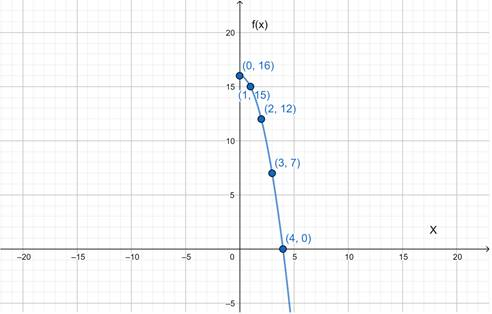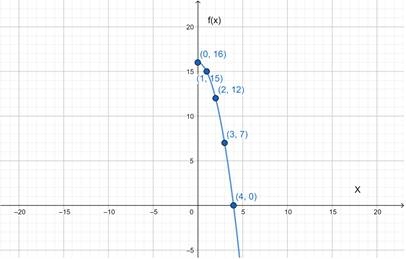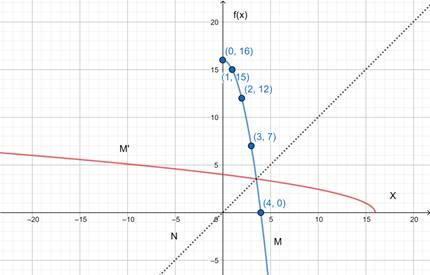# The graph of the function f ( x ) = 16 − x 2 , x ≥ 0### Precalculus: Mathematics for Calcu...

6th Edition
Stewart + 5 others
Publisher: Cengage Learning
ISBN: 9780840068071### Precalculus: Mathematics for Calcu...

6th Edition
Stewart + 5 others
Publisher: Cengage Learning
ISBN: 9780840068071

#### Solutions

Chapter 2.7, Problem 62E

a.

To determine

## To sketch: The graph of the function  f(x)=16−x2 , x≥0

Expert Solution

### Explanation of Solution

Given information:

f(x)=16x2 , x0

Graph:

 x 0 1 2 3 4 f(x) 16 15 12 7 0Interpretation: The graph is the function for f(x)=16x2 , x0 .

b.

To determine

Expert Solution

### Explanation of Solution

Given information:Graph:Interpretation:

Line M is the graph of function f . Line N is the graph of function y=x . After reflecting the line M in line N, we get line M' . Therefore, M' is the graph of function f1 .

c.

To determine

Expert Solution

f1(x)=16x

### Explanation of Solution

Given information: f(x)=16x2 , x0 .

Concept used: Let, f be a one-to-one function with domain A and range B. Then its inverse function f1 has domain B and range A and is defining by f1(y)=xf(x)=y for any y in B.

Calculation:

First, we replace f(x) with y ,

Then,

f(x)=16x2y=16x2y16=x2x2=16yx=16yf1(y)=16yf1(x)=16x

Thus, f1(x)=16x .

### Have a homework question?

Subscribe to bartleby learn! Ask subject matter experts 30 homework questions each month. Plus, you’ll have access to millions of step-by-step textbook answers!